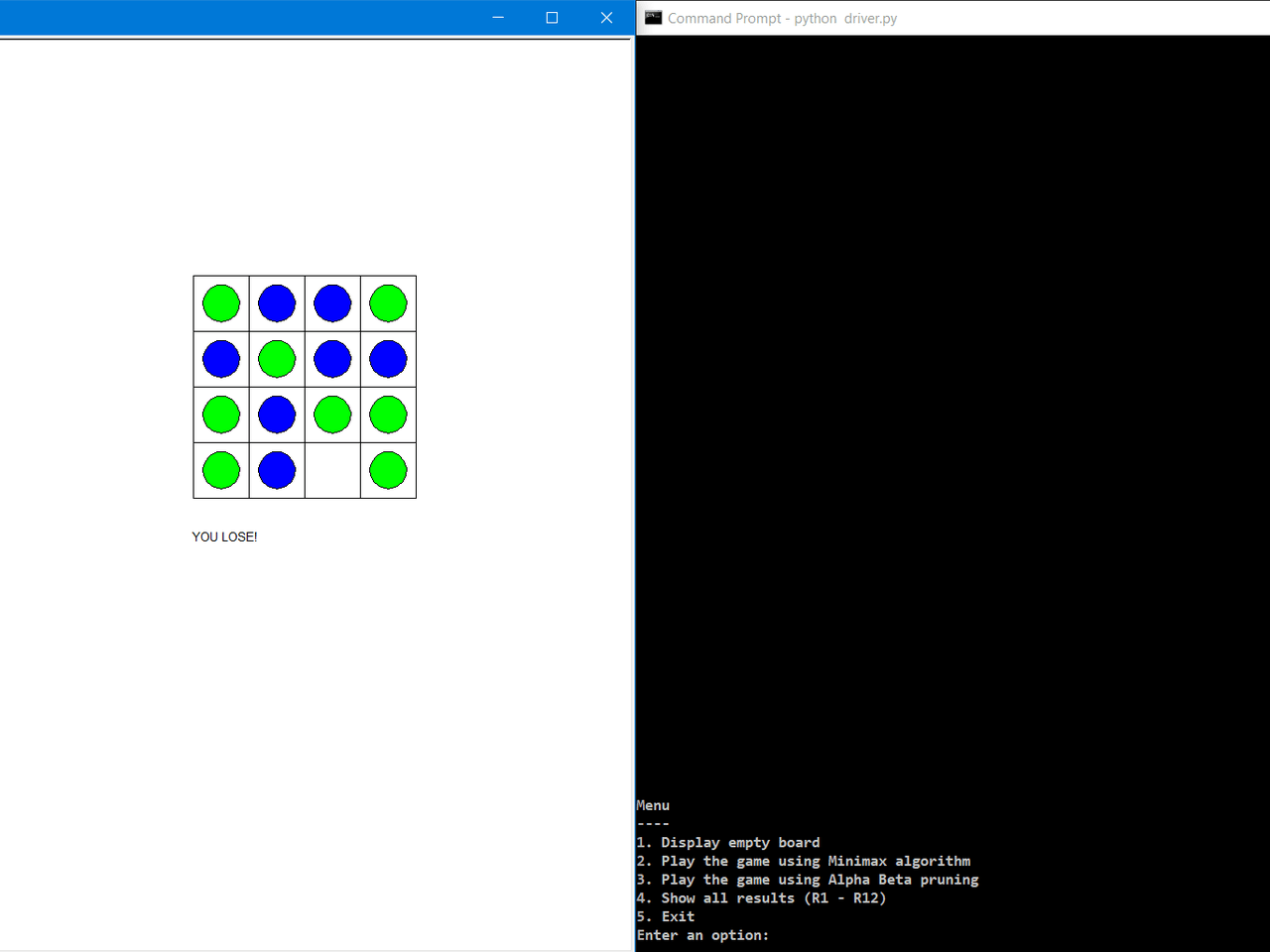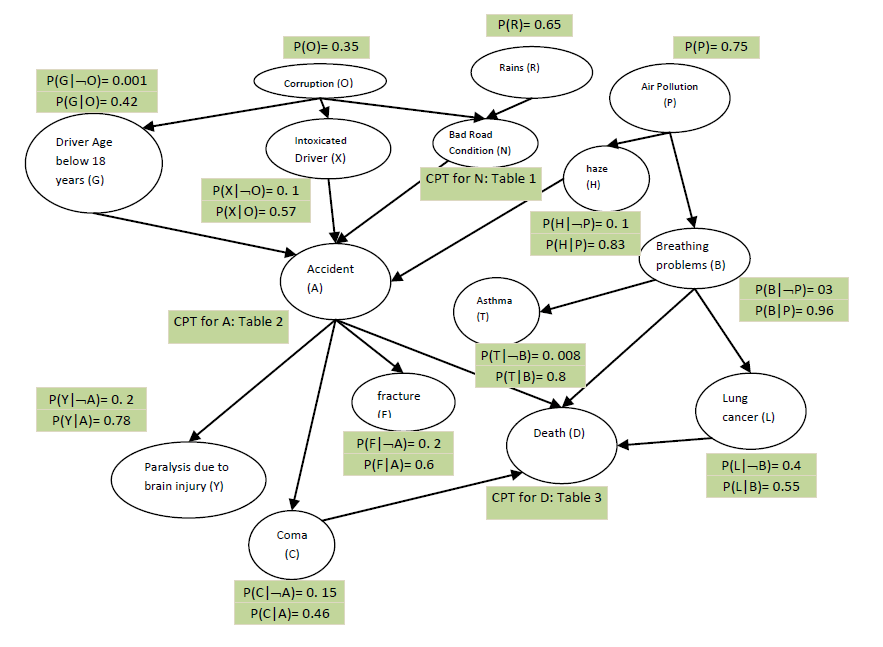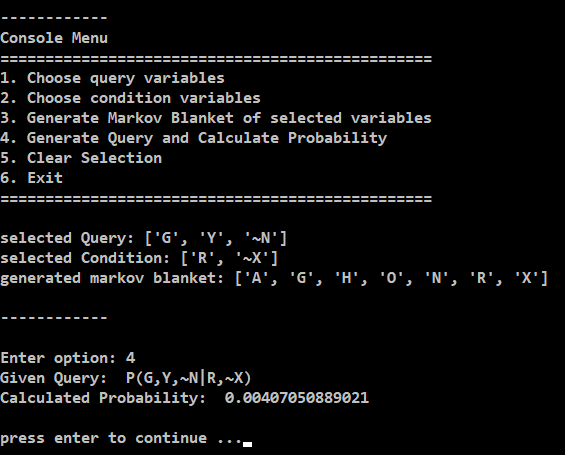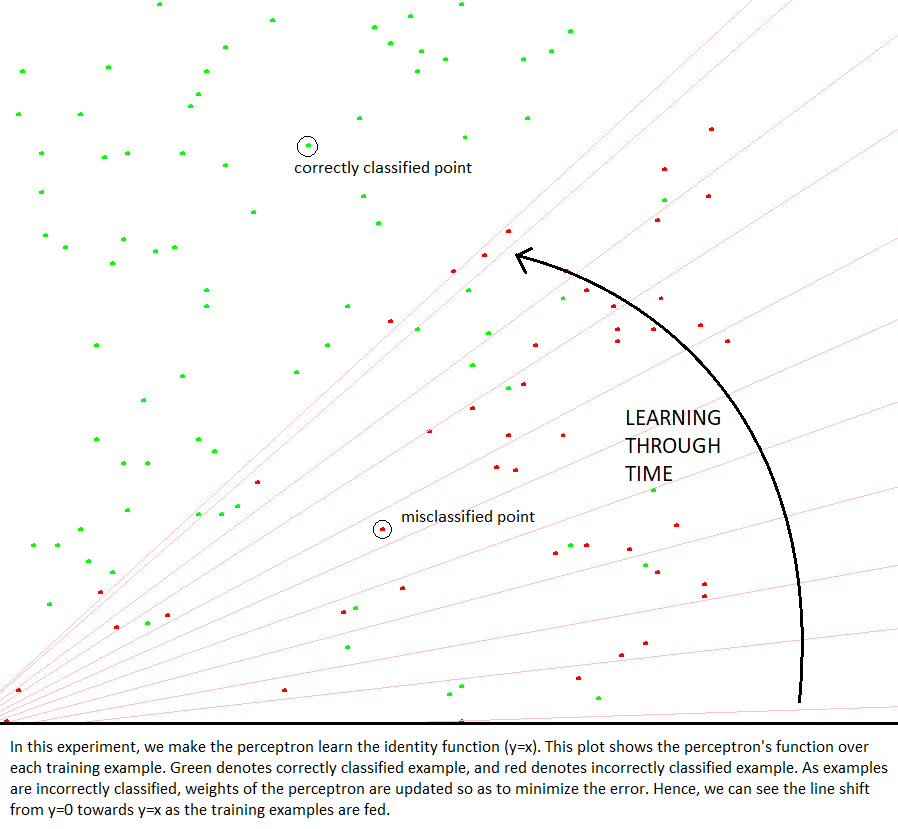## Deep Learning for Computer Vision – A Beginner’s Checklist

This document contains a list of resources to learn theoretical concepts in Machine Learning and to practice writing code online.

## Resources for some Stanford’s AI Courses

Here is a list of courses that are a part of Stanford’s AI Graduate Certificate, along with the resources (Slides / Notes / Lecture Videos etc.) for the courses.

Courses. (Required: CS221 and 3 elective courses from the rest)

• 4 units. CS221, CS224N, CS224U, CS228, CS229, CS230, CS231A, CS231N, AA228
• 3 units. CS157, CS223A, CS234, CS236, CS330

Prerequisites.

Course Materials

## Uniform sampling within an ellipsoid

Written for PyTorch with CUDA compatibility.

Use Case: Instead of doing a multivariate normal sampling (available in torch.distributions.multivariate_normal), one could also do a random sampling within a specified confidence region of the multivariate gaussian function.

Although it will be an approximation, one could obtain a confidence region using the variance across each dimension (diagonal of the covariance matrix). Suppose we define a 3*s boundary (Upto 3 x variance across each dimension is an accepted confidence interval), then EllipsoidSampler can be used to construct such an ellipsoid (with given mean = mu and lengths of axes = axes. This utility will further help sample from within the ellipsoid in a random fashion (instead of a random normal fashion).

Get code on gist.github

## Entropy, Cross-Entropy & KL-Divergence

Here is a 10-minute video by Aurélien Géron explaining entropy, cross-entropy and KL-divergence using Information Theory.

He has some more interesting videos on his channel. Do check it out!

## Connect-3 Game Playing Bot

We use Minimax algorithm to predict the next optimal move after every move by the user. This work demonstrates how a complete search done by Minimax algorithm can always yield optimal results. To speed up the search, alpha-beta prunning is implemented to prune moves that do no better than the currently explored moves. We test two methods using Minimax algorithm for game playing – with and without Alpha-Beta Prunning. A 14x faster first move is obtained using alpha-beta prunning.

The code is publicly available on github.## Probabilistic Inference using Bayesian Networks

In this work, we study the application of bayesian networks for probabilistic inference. We consider a hypothetical real-world scenario where we answer queries regarding various events (health problems, accidents etc.) caused by factors such as air pollution, bad road conditions etc.

Each event/factor is modeled as a random variable with a certain probability distribution function (given as input). Variable dependence graph is constructed and bayes rule is applied on the markov blanket of the query variables to reduce the computational effort. Detailed documentation can be found in the code.

The code is publicly available at github.## Visualizing Perceptron Learning

This program visualizes the learning process of a perceptron. For simplicity, we consider the perceptron to learn the identity function. We give a 2 dimensional input <x, y> and classify each point as being below the line or above the line (binary classification). We update the weights of the perceptron whenever misclassification occurs. Over several examples, the perceptron learns the identity mapping.

The code is publicly available on github.## The Curse of Dimensionality: Inside Out

The Curse of Dimensionality, introduced by Bellman, refers to the explosive nature of spatial dimensions and its resulting effects, such as, an exponential increase in computational effort, large waste of space and poor visualization capabilities. Higher number of dimensions theoretically allow more information to be stored, but practically rarely help due to the higher possibility of noise and redundancy in real world data. In this article, the effects of high dimensionality is studied through various experiments and the possible solutions to counter or mitigate such effects are proposed. The source code of the experiments performed is available publicly on github.

## Building an inline Turing Machine for C++

### Introduction

Turing Machines are mathematical abstractions of a computing systems. Suppose you are given an input string of letters of a given alphabet, and you want to do some computation on it, then given enough time and memory, a Turing Machine can do the job for you. The only caveat is, the language that you want to compute upon should be “computable”. What this means is, there are certain languages that are not computable in reasonable time by a Turing Machine. Such languages are called “hard”, because it is hard to write an algorithm to compute in reasonable time. This is a very abstract version of what a Turing Machine is. Let us explore it in brief. Continue reading